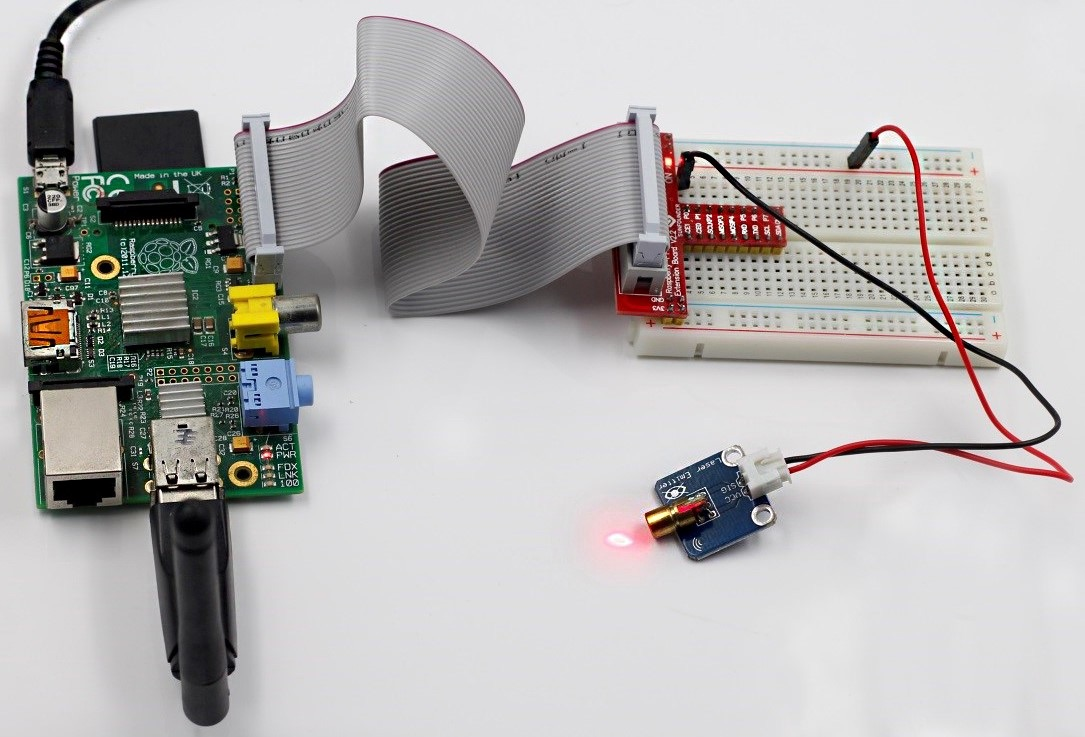## The plan

Our journey is made of 4 stations - each of them depending on the previous ones:

1. The tokenizer (aka “Lexical Analysis”): converting an input code - in `LISP` syntax - into an array of tokens.
2. The parser (aka “Syntactic Analysis”): transforming an array of tokens into an Abstract Syntax Tree (AST).
3. The emitter (aka “Code Generation”): string-ifying an AST into `C`-like code.
4. The compiler (aka “You made it”): combining all the pieces together.

(The interactive code snippets are powered by a tool of mine named KLIPSE.)

## Code generation

Now that we have our AST, it’s not so hard to transform the AST into code (if you are not afraid of recursions). This is the purpose of the `emitter`.

We are going to have an emitter per node type.## Simple node emitters

First, Let’s write the non-recursive emitters.

For `NumberLiteral` nodes - we emit the value as-is:

``````emitNumber = node => node.value
``````

Let’s see how it works:

``````emitNumber({
"type": "number",
"value": "2",
})
``````

For `StringLiteral` nodes - we wrap the node value with quotes:

``````emitString = node =>  `"\${node.value}"`
``````

Let’s see how it works:

``````emitString({
"type": "string",
"value": "Hello World!",
})
``````

## Recursive node emitters

And now the recursive emitters.

For the whole program - we emit the code of each expression appending a `;` and joining the expressions with `\n`:

``````emitProgram = node =>  node.body.map(exp => emitter(exp) + ";").join('\n');
``````

For an expression - this is where we deal with the facts that in `C`:

1. the function name comes before the parenthesis
2. the function arguments are comma separated
``````emitExpression = node =>
`\${node.name}(\${node.params.map(emitter).join(', ')})`
``````

## General node emitter

Combining all of it in the `emitter` - dispatching according to node type:

``````emitter = node => {
switch (node.type) {
case 'Program': return emitProgram(node);
case 'CallExpression': return emitExpression(node);
case 'NumberLiteral': return emitNumber(node);
case 'StringLiteral': return emitString(node);
default:
throw new TypeError(node.type);
}
}
``````

Let’s see how our `emitter` works with a simple expression:

``````emitter({
"type": "CallExpression",
"name": "subtract",
"params": [
{
"type": "NumberLiteral",
"value": "4"
},
{
"type": "NumberLiteral",
"value": "2"
}
]
})
``````

And now, let’s emit the code from the AST generated by our parser in the previous article:

``````ast = {
"type": "Program",
"body": [
{
"type": "CallExpression",
"name": "print",
"params": [
{
"type": "StringLiteral",
"value": "Hello"
},
{
"type": "NumberLiteral",
"value": "2"
}
]
},
{
"type": "CallExpression",
"params": [
{
"type": "NumberLiteral",
"value": "2"
},
{
"type": "CallExpression",
"name": "subtract",
"params": [
{
"type": "NumberLiteral",
"value": "4"
},
{
"type": "NumberLiteral",
"value": "2"
}
]
}
]
}
]
}
emitter(ast)
``````

Now, everything is set up for the last station:The compiler. No need to rest, you immediatly run towards it.